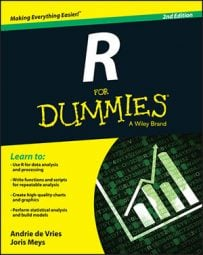##### R For DummiesAs time goes by, new data may appear and needs to be added to the dataset in R. Just like matrices, data frames can be appended using the rbind() function.

Say that Granny and Geraldine played another game with their team, and you want to add the number of baskets they made. The rbind() function lets you do that easily:

```> result <- rbind(baskets.df, c(7, 4))
> result
Granny Geraldine
1st   12     5
2nd   4     4
3rd   5     2
4th   6     4
5th   9    12
6th   3     9
7    7     4```

The data frame result now has an extra observation compared to baskets.df. rbind() can take multiple arguments, as long as they’re compatible. In this case, you bind a vector c(7, 4) at the bottom of the data frame.

Note that R, by default, sets the row number as the row name for the added rows. You use the rownames() function to adjust this, or you can immediately specify the row name between quotes in the rbind() function:

`> baskets.df <- rbind(baskets.df, "7th" = c(7, 4))`

Note that you must use quotation marks around 7th, because it starts with a number. Without quotation marks, R doesn’t recognize it as a name. If you check the object baskets.df now, you see the extra observation at the bottom with the correct row name:

```> baskets.df
Granny Geraldine
1st   12     5
2nd   4     4
3rd   5     2
4th   6     4
5th   9    12
6th   3     9
7th   7     4```

Alternatively, you can use indexing to add an extra observation. Keep reading to see how.

## Adding a series of new observations using rbind

If you need to add multiple new observations to a data frame, doing it one-by-one is not entirely practical. Luckily, you can use rbind() to attach a matrix or a data frame with new observations to the original data frame. The matching of the columns is done by name, so you need to make sure that the columns in the matrix or the variables in the data frame with new observations match the variable names in the original data frame.

Let’s add another two game results to the data frame baskets.df. First, you construct a new data frame with the number of baskets Granny and Geraldine scored, like this:

`> new.baskets <- data.frame(Granny = c(3, 8), Geraldine = c(9, 4))`

If you use the data.frame() function to construct a new data frame, you can immediately set the variable names by specifying them in the function call, as in the preceding example. That code creates a data frame with the variables Granny and Geraldine where each variable contains the vector given after the equal sign.

To be able to bind the data frame new.baskets to the original baskets.df, you have to make sure that the variable names match exactly, including the case.

Next, you add the optional row names and the necessary column names with the following code:

`> rownames(new.baskets) <- c("8th", "9th")`

To add the matrix to the data frame, you simply do the following:

`> baskets.df <- rbind(baskets.df, new.baskets)`

You can try yourself to do the same thing using a data frame instead of a matrix.

## Adding a series of values using indices

You also can use the indices to add a set of new observations at one time. You get exactly the same result if you change all the previous code by this simple line:

`> baskets.df[c("8th", "9th"), ] <- matrix(c(3, 8, 9, 4), ncol = 2)`

With this code, you do the following:

• Create a matrix with two columns.

• Create a vector with the row names 8th and 9th.

• Use this vector as row indices for the data frame baskets.df.

• Assign the values in the matrix to the rows with names 8th and 9th. Because these rows don’t exist yet, R creates them automatically.

Actually, you don’t need to construct the matrix first; you can just use a vector instead. Exactly as with matrices, data frames are filled column-wise. So, the following code gives you exactly the same result:

`> baskets.df[c("8th", "9th"), ] <- c(3, 8, 9, 4)`

This process works only for data frames, though. If you try to do the same thing with matrices, you get an error. In the case of matrices, you can only use indices that exist already in the original object.

You have multiple equally valid options for adding observations to a data frame. Which option you choose depends on your personal choice and the situation. If you have a matrix or data frame with extra observations, you can use rbind(). If you have a vector with row names and a set of values, using the indices may be easier.